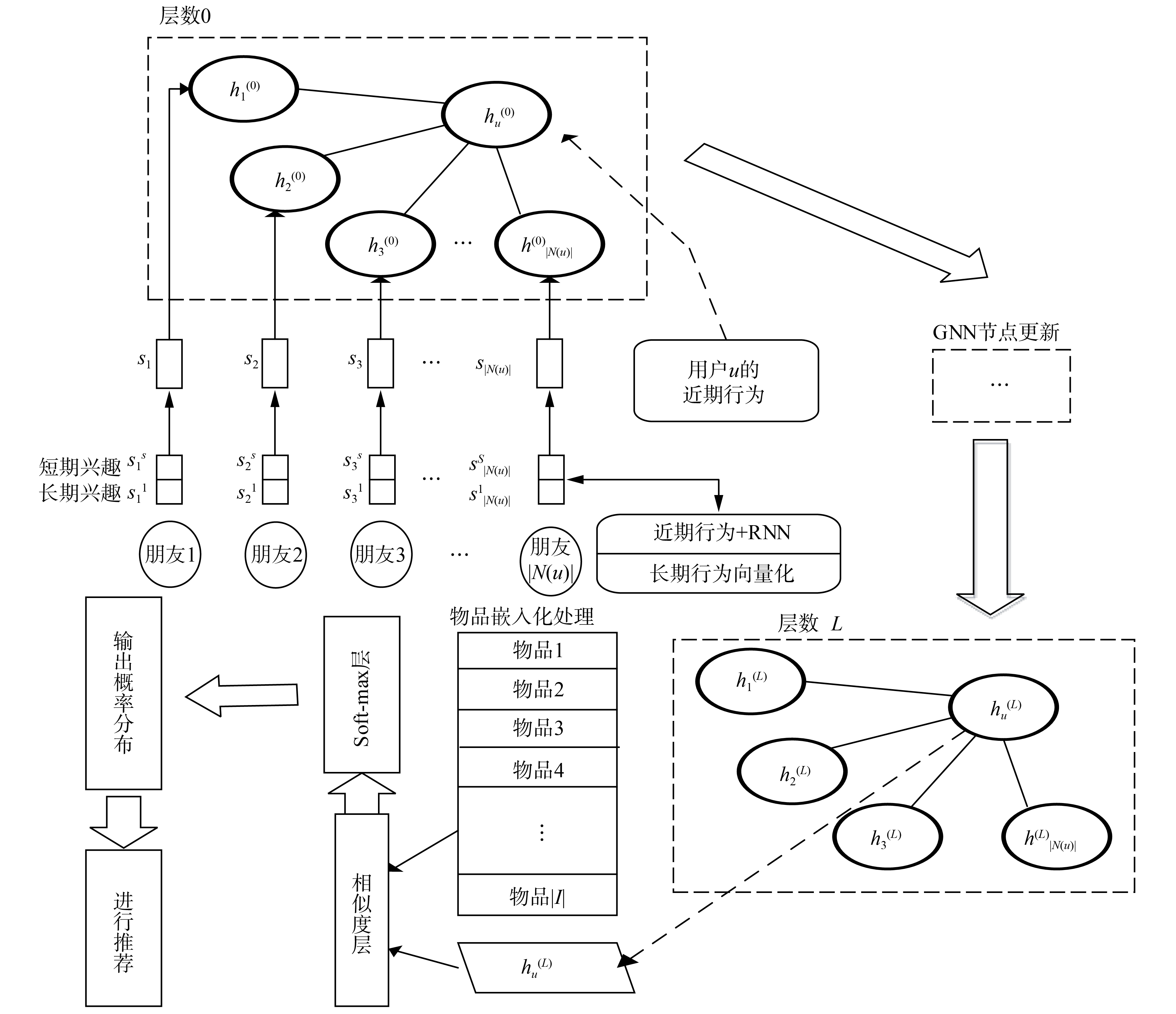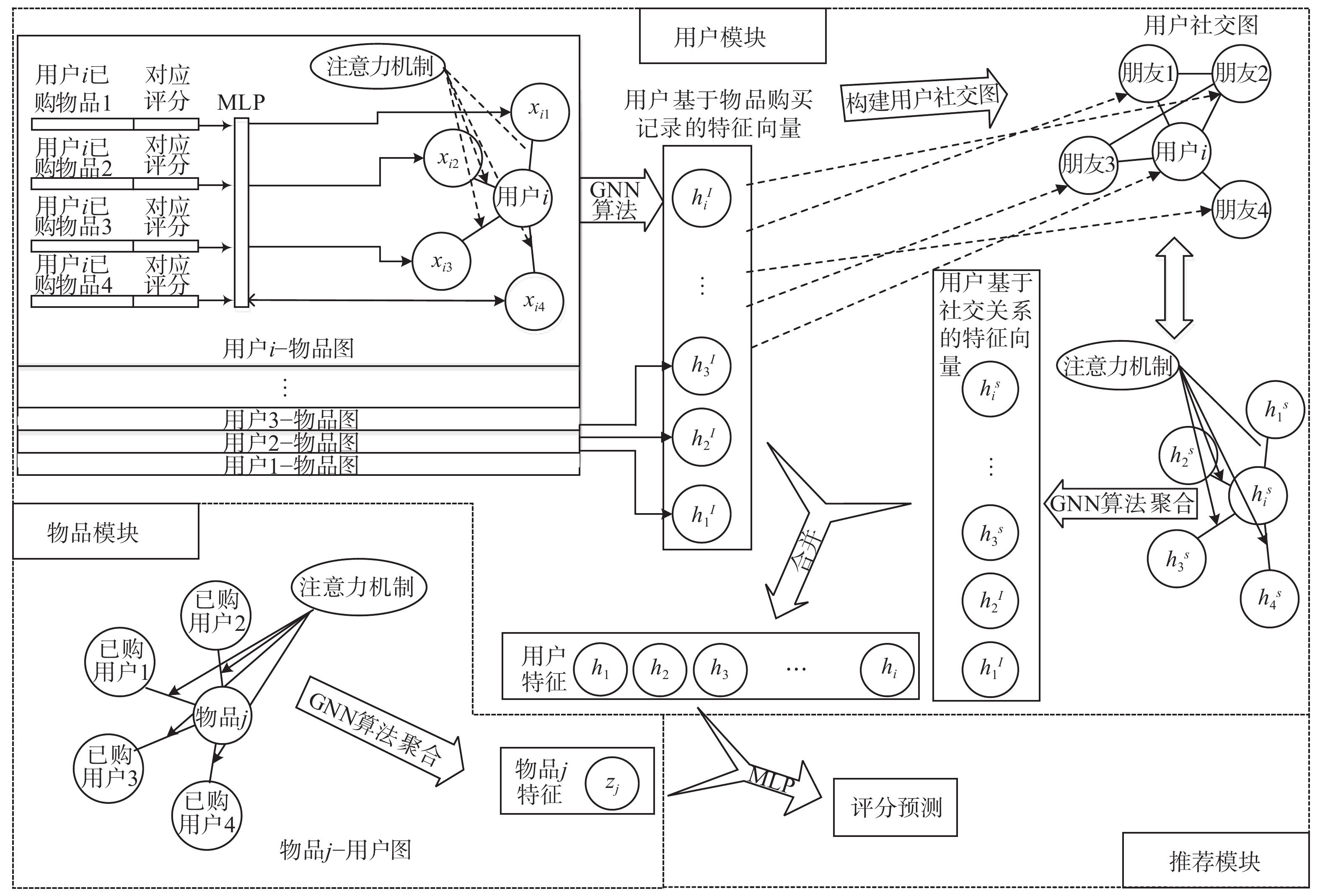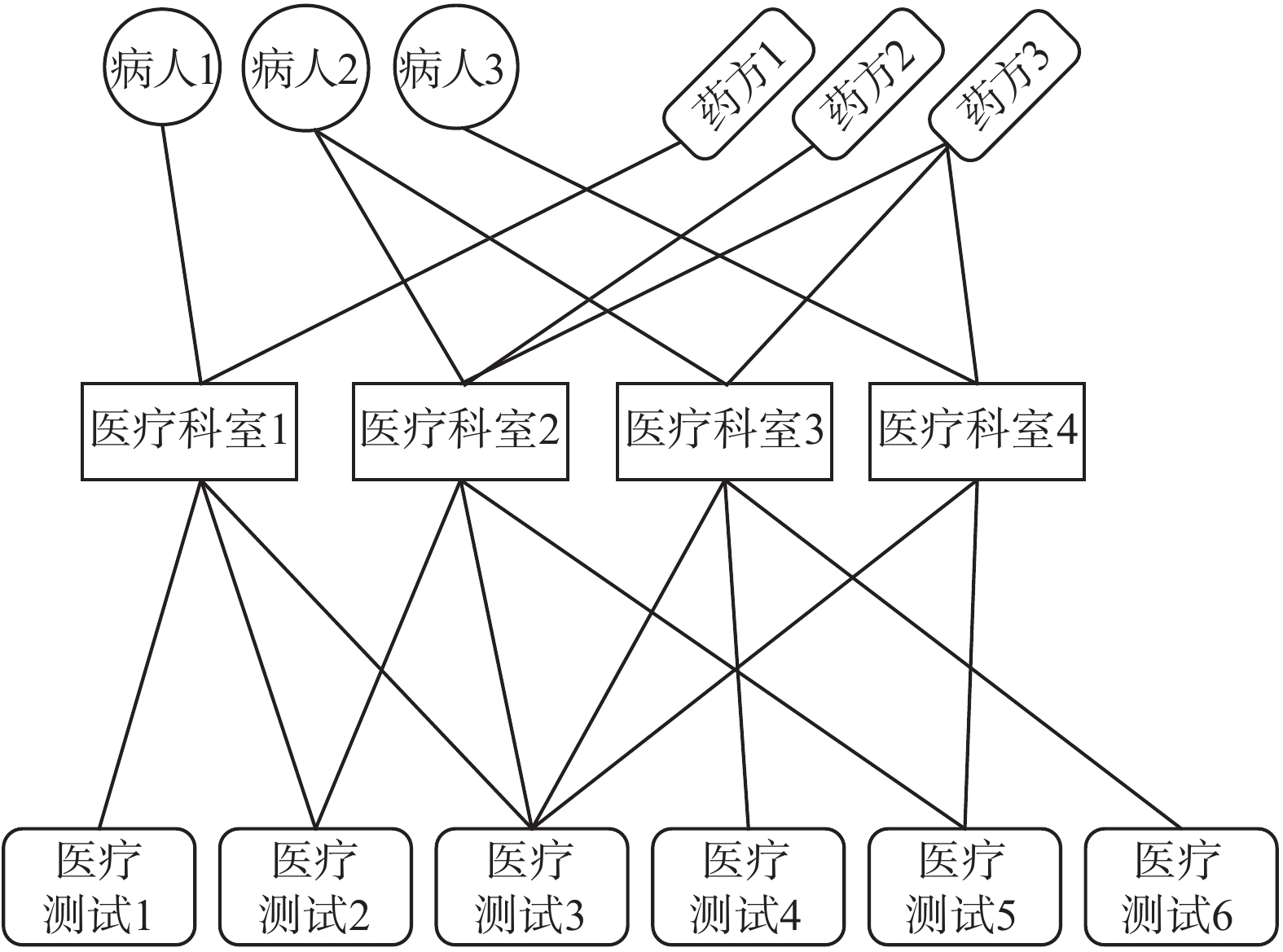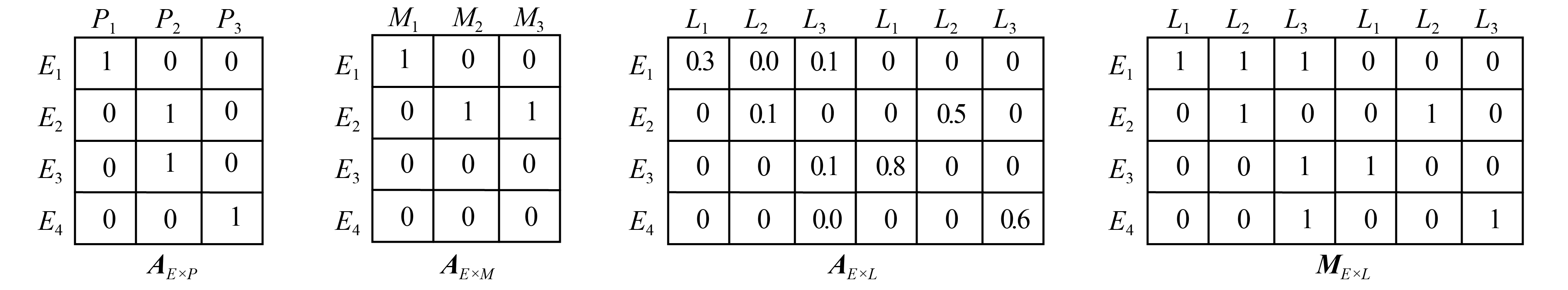﻿ 图神经网络推荐研究进展
«上一篇文章快速检索 高级检索

 智能系统学报2020, Vol. 15Issue (1): 14-24  DOI: 10.11992/tis.2019080340

### 引用本文WU Guodong, ZHA Zhikang, TU Lijing, et al. Research advances in graph neural network recommendation[J]. CAAI Transactions on Intelligent Systems, 2020, 15(1): 14-24. DOI: 10.11992/tis.201908034.### 文章历史

1. 东华大学 管理学院，上海 200051;
2. 安徽农业大学 信息与计算机学院，安徽 合肥 230036

Research advances in graph neural network recommendation
WU Guodong 1,2, ZHA Zhikang 2, TU Lijing 2, TAO Hong 2, SONG Fugeng 1
1. School of Business and Management, Donghua University, Shanghai 200051, China;
2. School of Information and Computer, Anhui Agricultural University, Hefei 230036, China
Abstract: Graph neural network (GNN) has the advantage of feature extraction and representation of data from the field of Graph. In recent years, it has become a hotspot of artificial intelligence research, and the recommendation of Graph Neural Network is also a new direction of recommendation system research. Based on the in-depth study of GNN model, this paper analyzes the GNN recommendation process, and discusses in detail the main progress and deficiencies of GNN recommendation studies from three aspects: undirected unit graph recommendation, undirected binary graph recommendation and undirected multivariate graph recommendation. The main difficulties in existing GNN recommendation studies are clarified, and the research directions of GNN recommendation in the future, including GNN contextual recommendation, GNN cross-domain recommendation, GNN group recommendation, and GNN recommendation’s interpretability, and so on, are pointed out in the end.
Key words: graph neural network    recommendation system    deep learning    entity relations    society relation    collaborative filtering    undirected graph    directed graph

1 图神经网络

 ${{{h}}_{{v}}} = f\left( {{{{X}}_{{v}}},{{{X}}_{{\rm{CO}}\left[ {{v}} \right]}},{{{h}}_{{\rm{ne}}\left[ {{v}} \right]}},{{{X}}_{{\rm{ne}}\left[ {{v}} \right]}}} \right)$ (1)
 ${{{o}}_{{v}}} = g\left( {{{{h}}_{{v}}},{{{X}}_{{v}}}} \right)$ (2)

 $H = F(H,X)$ (3)
 $O = G(H,{X_N})$ (4)

 ${{{H}}^{{{t}} + 1}} = {{F}}\left( {{{{H}}^{{t}}},{{X}}} \right)$ (5)

GNN有多种变体，如根据训练方法的差异有GraphSAGE、FastGCN、ControlVariate、Co-training GCN和Self-training GCN等；根据信息传播计算方式的不同有Spectral Network、MoNet、DCNN、Gate Graph Neural Networks、Sentence LSTM等。

1)有向图GNN

 ${{{H}}^t} = {\rm{\sigma }}({{D}}_p^{ - 1}{{{A}}_p} \cdot {\rm{\sigma }}({{D}}_c^{ - 1}{{{A}}_c}{{{H}}^{t - 1}}{{{W}}_c}){{{W}}_p})$ (6)

2)无向图GNN

2 推荐系统

3 GNN推荐主要过程

4 GNN推荐相关研究

4.1 无向单元图GNN推荐

1)将用户近期的浏览内容，通过循环神经网络(RNN)得到表示用户兴趣的hn，并用hn描述用户的节点信息；

2)将朋友的近期行为记录，通过RNN得到sks，用来表示该朋友的短期兴趣；

3)将朋友长期行为向量化，得skl，见式(7)：

 ${{s}}_{{k}}^{{l}} = {W_u}\left[ {k,:} \right]$ (7)

4)将上述两个向量联合起来，构成代表目标节点用户的嵌入化向量 ${{{s}}_k}$ ，见式(8)：

 ${{{s}}_{{k}}} = {\rm{ReLU}}\left( {{W_1}\left[ {{{s}}_{{k}}^{{s}},{{s}}_{{k}}^{{l}}} \right]} \right)$ (8)

5)目标节点的初始状态选取为 ${h_n}$ ，邻居节点的初始状态选取为 ${s_k}$ ，利用构建完成的用户与好友之间关系的拓扑图，通过计算每层的目标节点和邻居节点的相似度关系，提取出注意力系数 ${a_{uk}}$ ，见式(9)：

 ${\rm{\alpha }}_{uk}^{\left( {\rm{l}} \right)} = \frac{{{\rm{exp}}\left( {f\left( {{{h}}_{{u}}^{\left( {{l}} \right)},{{h}}_{{k}}^{\left( {{l}} \right)}} \right)} \right)}}{\sum \nolimits_{j \in N\left( u \right) \cup \left\{ u \right\}} {\rm{\alpha }}_{uk}^{\left( l \right)}{{h}}_{{k}}^{\left( {{l}} \right)}}$ (9)

6)将所有的邻居节点信息通过注意力系数相加后，得到表示目标节点所有邻居节点的信息向量 ${{{\hat h}}_{{u}}}$ ，见式(10)，再将 ${\hat {{h}}_u}$ 经过偏置加权之后作为节点的下一轮状态进行更新，见式(11)：

 ${{{\hat h}}_{{u}}} = \sum\nolimits_{k \in N\left( u \right) \cup \left\{ u \right\}} {{\rm{\alpha }}_{uk}^{\left( l \right)}{{h}}_{{k}}^{\left( {{l}} \right)}}$ (10)
 $h_{{u}}^{\left( {{{l}} + 1} \right)} = {\rm{ReLU}}\left( {{{{W}}^{\left( {{l}} \right)}}{{\hat h}}_{{u}}^{\left( {{l}} \right)}} \right)$ (11)

7)将节点的特征与嵌入化得到的物品特征进行相似度运算，通过softmax进行输出，此时输出的结果就是某用户对物品可能的感兴趣程度。Download: 图 1 无向单元图GNN推荐模型 Fig. 1 Single-unit undirected graph GNN model

4.2 无向二元图GNN推荐Download: 图 2 基于社交关系的GraphRec模型 Fig. 2 Social-based recommendation model-GraphRec

1)对所提取的物品qa向量和对应的评分er向量连接处理，通过一个多层感知器得到xia向量(其中a表示用户购买的相关物品，i表示某一个用户)，见式(12)：

 ${{{x}}_{{{ia}}}} = {{{g}}_{{v}}}\left( {\left[ {{{{q}}_a} \oplus {{{e}}_r}} \right]} \right)$ (12)

2)将计算出的xia和用户pi通过一个两层的神经网络得到该用户对物品的注意力系数α*，见式(13)：

 $\alpha _{ia}^* = {{W}}_2^{{\rm{T}}} \cdot \sigma \left( {{{{W}}_1} \cdot \left[ {{{{x}}_{{{ia}}}} \oplus {{{p}}_{{i}}}} \right] + {{{b}}_1}} \right) + {{{b}}_2}$ (13)

3)对 ${\alpha ^{\rm{*}}}$ 进行 ${\rm{soft}}\max$ 处理，得到 ${\alpha _{ia}}$ 作为最终的注意力系数，见式(14)：

 ${{{\alpha}} _{{{ia}}}} = \frac{{{\rm{exp}}\left( {{{\alpha}} _{{{ia}}}^*} \right)}}{{\displaystyle\sum \nolimits_{{{a}} \in {{C}}\left( {{i}} \right)} {\rm{exp}}\left( {{{\alpha}} _{{{ia}}}^*} \right)}}$ (14)

4)用αia对之前得到的xia向量进行加权，并且通过一个多层感知器(GNN的简单聚合函数)得到用户−物品特征hi，见式(15)：

 ${{h}}_{{i}}^{{I}} = {\rm{\sigma}} ({{w}} \cdot \left\{ {\left. {\sum\nolimits_{a \in c(i)} {{a_{ia}}{x_{ia}}} } \right\}} \right. + b)$ (15)

5)用同样的方法对该用户的好友进行处理，得到对应hio(o表示用户好友集i的一个好友)；

6)使用2)中的方法对 ${h_{io}}$ 和用户向量 ${p_i}$ 通过一个两层神经网络提取注意力系数β*，见式(16)，并且通过softmax进行处理可以得到β，见式(17)：

 $\beta _{io}^* = {{W}}_2^{\rm{T}} \cdot \sigma \left( {{{{W}}_1} \cdot \left[ {{{h}}_{{o}}^{\rm{I}} \oplus {{{p}}_{{i}}}} \right] + {{{b}}_1}} \right) + {{{b}}_2}$ (16)
 ${\beta _{io}} = \frac{{{\rm{exp}}\left( {\beta _{i0}^*} \right)}}{{\displaystyle\sum \nolimits_{o \in N\left( i \right)} {\rm{exp}}\left( {\beta _{io}^*} \right)}}$ (17)

7)将得到的βhi进行加权并通过一个多层感知器，最后得到具有刻画用户i社交关系的向量 ${{h}}_i^S$ ，见式(18)：

 ${{h}}_i^S = {\rm{\sigma}} ({{w}} \cdot \left\{ {\left. {\sum\nolimits_{o \in N(i)} {{\beta _{io}}h_o^I} } \right\}} \right.{{ + b)}}$ (18)

 ${\rm{Loss}} = \frac{1}{{2\left| O \right|}}\mathop \sum \limits_{i,j \in O} {\left( {{{r'}_{ij}} - {r_{ij}}} \right)^2}$ (19)

4.3 无向多元图GNN推荐Download: 图 3 患者医疗诊断关系和医疗测试结果 Fig. 3 The graph of patients’ consults relation and laboratory testing resultsDownload: 图 4 病患、医疗科室、药方以及医疗测试结果关系矩阵 Fig. 4 Relation matrix between patients encounters medications and laboratory test data

 ${{{H}}^{\left( {{{k}} + 1} \right)}}\left( {{v}} \right) = f_1^{{{W}}_1^{\left( {{k}} \right)}}\left( {{{{H}}^{\left( {{k}} \right)}}\left( {{v}} \right),f_2^{{{W}}_2^{\left( {{k}} \right)}}\left( {\left\{ {{{{H}}^{\left( {{k}} \right)}}\left( w \right)|w \in N\left( v \right)} \right\}} \right)} \right)$ (20)

Mao对激活函数 ${\phi}$ 使用了最简单的求和函数，如式(21)所示，对节点类型为i的节点的第k轮计算，其中hj表示他的连接节点。

 ${{H}}_{{i}}^{\left( {{{k}} + 1} \right)} = \phi \left( { \mathop \sum \limits_{j = 0}^n {{{A}}_{{{ij}}}} \cdot {{H}}_{{j}}^{\left( {{k}} \right)} \cdot {{W}}_{{j}}^{\left( {{k}} \right)}} \right)$ (21)

 $L = {L_m}\left( {{{P}},{{{A}}_{{{E}} \times {{M}}}}} \right) + \lambda {L_L}\left( {{{V}},{{{A}}_{{{E}} \times {{L}}}}} \right)$ (22)
 ${L_M}\left( {P,A} \right) = - \frac{1}{{{N_E}}}\mathop \sum \limits_i^{{N_E}} \mathop \sum \limits_j^{{N_M}} \frac{{{N_n}}}{{{N_p}}}{a_{ij}}\log {p_{ij}} + \left( {1 - {a_{ij}}} \right)\log \left( {1 - {p_{ij}}} \right)$ (23)
 ${L_L}\left( {V,A} \right) = - \frac{1}{{{N_E}}}\mathop \sum \limits_i^{{N_E}} \mathop \sum \limits_j^{{N_L}} {m_{ij}}{\left( {{v_{ij}} - {a_{ij}}} \right)^2}$ (24)

5 现有GNN推荐研究难点 5.1 多源多模态信息的GNN推荐问题

5.2 GNN的有向图推荐问题

5.3 GNN的动态推荐问题

5.4 GNN网络更新层数问题

5.5 数据稀疏问题

GNN推荐中，通过构建节点和边，将各个对象用边“连接”起来，在缓解数据稀疏性方面相比传统的推荐模型会有提升。但GNN推荐模型在面临数据稀疏的时候，往往会出现两个问题：1)如果节点对应的对象没有任何相关记录时，相关推荐算法将难以实现，如文献中，如果有新来的病人或者病人的就诊记录很少，如仅为一条记录，GNN推荐模型会难以得到可以代表用户特征的最终结果；2)节点属性维度缺失问题。一个向量化的多维节点，每一个维度都反映一个对应的属性，如果该属性的值是未知的，在向量化的过程中就无法写入相应的数值，将会影响到推荐的效果。在文献中就出现了病人未参加部分医疗测试的情况，作者通过重新构建测试结果，解决这个问题，但模型的复杂度也随之增加。

6 GNN推荐未来研究方向

1)基于上下文的GNN推荐

2)基于GNN的跨领域推荐

3)基于GNN的群组推荐

4)基于GNN的套餐推荐

5) GNN推荐与其他推荐方法融合

6) GNN推荐的可解释性研究

GNN的应用范围很广泛，比如文献利用GNN模拟各个物理系统间的关系，进行演绎来预测整个系统的下一个状态，这说明GNN具有一定的因果推理能力。现有GNN推荐研究中，尽管取得了不错的推荐效果，但是推荐结果往往很难给出一个令用户信服的科学解释。如在文献中，作者使用多个GNN模型得出特征后，通过MLP进行评分预测，仍然缺乏一定的可解释性。

7 结束语

  ZHOU J, CUI G, ZHANG Z, et al. Graph neural networks: a review of methods and applications[J]. arXiv: 1812.08434, 2018. (0)  BASTINGS J, TITOV I, AZIZ W, et al. Graph convolutional encoders for syntax-aware neural machine translation[C]//Proceedings of the 2017 Conference on Empirical Methods in Natural Language Processing. Copenhagen, Denmark, 2017: 1957−1967. (0)  HENAFF M, BRUNA J, LECUN Y. Deep convolutional networks on graph-structured data[J]. arXiv:1506.05163, 2015. (0)  ZHANG Yuhao, QI Peng, MANNING C D. Graph convolution over pruned dependency trees improves relation extraction[C]//Proceedings of the 2018 Conference on Empirical Methods in Natural Language Processing. Brussels, Belgium, 2018: 2205−2215. (0)  WANG Xiaolong, YE Yufei, GUPTA A. Zero-shot recognition via semantic embeddings and knowledge graphs[C]//Proceedings of 2018 IEEE/CVF Conference on Computer Vision and Pattern Recognition. Salt Lake City, USA, 2018: 6857−6866. (0)  RHEE S,SEO S, KIM S.Hybrid approach of relation network and localized graph convolutional filtering for breast cancer subtype classification[J].arXiv: 1711.05859,2017. (0)  KAWAMOTO T, TSUBAKI M, OBUCHI T. Mean-field theory of graph neural networks in graph partitioning[C]//Proceedings of the 32nd International Conference on Neural Information Processing Systems. Red Hook, USA, 2018: 4361−4371. (0)  HAMILTON W, YING Zhotao, LESKOVEC J. Inductive representation learning on large graphs[C]//Advances in Neural Information Processing Systems. Long Beach, US, 2017: 1024−1034. (0)  CHEN J, MA T, XIAO C. Fastgcn: fast learning with graph convolutional networks via importance sampling[J]. arXiv:1801.10247, 2018. (0)  CHEN Jianfei, ZHU Jun, SONG Le. Stochastic training of graph convolutional networks with variance reduction[C]//Proceedings of the 35th International Conference on Machine Learning. Stockholm, Sweden, 2018: 941−949. (0)  LI Q, HAN Z, WU X M. Deeper insights into graph convolutional networks for semi-supervised learning[C]//Thirty-Second AAAI Conference on Artificial Intelligence. New Orleans, USA,2018. (0)  BRUNA J, ZAREMBA W, SZLAM A, et al. Spectral networks and locally connected networks on graphs[C]//International Conference on Learning Representations (ICLR2014). Banff, Canada, 2014: 1−14. (0)  MONTI F, BOSCAINI D, MASCI J, et al. Geometric deep learning on graphs and manifolds using mixture model CNNs[C]//Proceedings of 2017 IEEE Conference on Computer Vision and Pattern Recognition. Honolulu, USA, 2017: 5115−5124. (0)  ATWOOD J, TOWSLEY D. Diffusion-convolutional neural networks[C]//Advances in Neural Information Processing Systems. Barcelona, Spain, 2016: 1993−2001. (0)  LI Y, ZEMEL R, BROCKSCHMIDT M, et al. Gated graph sequence neural networks [J]. arXiv:1511.05493, 2015. (0)  ZHANG Yue, LIU Qi, SONG Linfeng. Sentence-state LSTM for text representation[C]//Proceedings of the 56th Annual Meeting of the Association for Computational Linguistics. Melbourne, Australia, 2018: 317−327. (0)  KAMPFFMEYER M, CHEN Yinbo, LIANG Xiaodan, et al. Rethinking knowledge graph propagation for zero-shot learning[C]//Proceedings of 2019 IEEE/CVF Conference on Computer Vision and Pattern Recognition. Long Beach, USA, 2019: 11487−11496. (0)  黄璐, 林川杰, 何军, 等. 融合主题模型和协同过滤的多样化移动应用推荐[J]. 软件学报, 2017, 28(3): 708-720. HUANG Lu, LIN Chuanjie, HE Jun, et al. Diversified mobile app recommendation combining topic model and collaborative filtering[J]. Journal of software, 2017, 28(3): 708-720. (0)  胡堰, 彭启民, 胡晓惠. 一种基于隐语义概率模型的个性化Web服务推荐方法[J]. 计算机研究与发展, 2014, 51(8): 1781-1793. HU Yan, PENG Qimin, HU Xiaohui. A personalized Web service recommendation method based on latent semantic probabilistic model[J]. Journal of computer research and development, 2014, 51(8): 1781-1793. DOI:10.7544/issn1000-1239.2014.20130024 (0)  刘建勋, 石敏, 周栋, 等. 基于主题模型的Mashup标签推荐方法[J]. 计算机学报, 2017, 40(2): 520-534. LIU Jianxun, SHI Min, ZHOU Dong, et al. Topic model based tag recommendation method for Mashups[J]. Chinese journal of computers, 2017, 40(2): 520-534. DOI:10.11897/SP.J.1016.2017.00520 (0)  曹俊豪, 李泽河, 江龙, 等. 一种融合协同过滤和用户属性过滤的混合推荐算法[J]. 电子设计工程, 2018, 26(9): 60-63. CAO Junhao, LI Zehe, JIANG Long, et al. A hybrid recommendation algorithm based on collaborative filtering and user attribute filtering[J]. Electronic design engineering, 2018, 26(9): 60-63. DOI:10.3969/j.issn.1674-6236.2018.09.014 (0)  张双庆. 一种基于用户的协同过滤推荐算法[J]. 电脑知识与技术, 2019, 15(1): 19-21. ZHANG Shuangqing. User-based collaborative filtering recommendation algorithm[J]. Computer knowledge and technology, 2019, 15(1): 19-21. (0)  邓园园, 吴美香, 潘家辉. 基于物品的改进协同过滤算法及应用[J]. 计算机系统应用, 2019, 28(1): 182-187. DENG Yuanyuan, WU Meixiang, PAN Jiahui. Improved item-based collaborative filtering algorithm and its application[J]. Computer systems & applications, 2019, 28(1): 182-187. DOI:10.15888/j.cnki.csa.006726 (0)  高玉凯, 王新华, 郭磊, 等. 一种基于协同矩阵分解的用户冷启动推荐算法[J]. 计算机研究与发展, 2017, 54(8): 1813-1823. GAO Yukai, WANG Xinhua, GUO Lei, et al. Learning to recommend with collaborative matrix factorization for new users[J]. Journal of computer research and development, 2017, 54(8): 1813-1823. DOI:10.7544/issn1000-1239.2017.20170188 (0)  王伟, 陈伟, 祝效国, 等. 众筹项目的个性化推荐: 面向稀疏数据的二分图模型[J]. 系统工程理论与实践, 2017, 37(4): 1011-1023. WANG Wei, CHEN Wei, ZHU K, et al. Personalized recommendation of crowd-funding campaigns: a bipartite graph approach for sparse data[J]. Systems engineering–theory & practice, 2017, 37(4): 1011-1023. DOI:10.12011/1000-6788(2017)04-1011-13 (0)  ELKAHKY A M, SONG Yang, HE Xiaodong. A multi-view deep learning approach for cross domain user modeling in recommendation systems[C]//Proceedings of the 24th International Conference on World Wide Web. Florence, Italy, 2015: 278−288. (0)  ZHENG Lei, NOROOZI V, YU P S. Joint deep modeling of users and items using reviews for recommendation[C]//Proceedings of the Tenth ACM International Conference on Web Search and Data Mining. New York, USA, 2017: 425−434. (0)  LIU Q, WU S, WANG L, et al. Predicting the next location: a recurrent model with spatial and temporal contexts[C]//Proceedings of the Thirtieth AAAI Conference on Artificial Intelligence. Arizona, USA ,2016: 194−200. (0)  XU,KEYULU,et al.How powerful are graph neural networks [J]. arXiv:1810.00826, 2018. (0)  SONG Weiping, XIAO Zhiping, WANG Yifan, et al. Session-based social recommendation via dynamic graph attention networks[C]//Proceedings of the Twelfth ACM International Conference on Web Search and Data Mining. New York, United States, 2019: 555−563. (0)  FAN Wenqi, MA Yao, LI Qing, et al. Graph neural networks for social recommendation[C]//The World Wide Web Conference. New York, USA, 2019: 417−426. (0)  YING R, HE Ruining, CHEN Kaifeng, et al. Graph convolutional neural networks for web-scale recommender systems[C]//Proceedings of the 24th ACM SIGKDD International Conference on Knowledge Discovery & Data Mining. New York, USA, 2018: 974−983. (0)  CUI Zeyu, LI Zekun, WU Shu, et al. Dressing as a whole: outfit compatibility learning based on node-wise graph neural networks[C]//The World Wide Web Conference. New York, USA, 2019: 307−317. (0)  WANG X, HE X, WANG M, et al. Neural graph collaborative filtering[J]. arXiv:1905.08108, 2019. (0)  WANG Hongwei, ZHAO Miao, XIE Xing, et al. Knowledge graph convolutional networks for recommender systems[C]//The World Wide Web Conference. San Francisco, USA, 2019: 3307−3313. (0)  MAO C, YAO L, LUO Y. MedGCN: Graph convolutional networks for multiple medical tasks[J]. arXiv: 1904.00326, 2019. (0)  刘云, 王颖, 亓国涛, 等. 时间上下文的协同过滤Top-N推荐算法[J]. 计算机技术与发展, 2017, 27(7): 79-82. LIU Yun, WANG Ying, QI Guotao, et al. Collaborative filtering top-N recommendation algorithm based on time context[J]. Computer technology and development, 2017, 27(7): 79-82. DOI:10.3969/j.issn.1673-629X.2017.07.019 (0)  沈记全, 王磊, 侯占伟, 等. 基于情景上下文与信任关系的旅游景点推荐算法[J]. 计算机应用研究, 2018, 35(12): 3640-3643. SHEN Jiquan, WANG Lei, HOU Zhanwei, et al. Attractions recommendation algorithm based on situational context and trust relationship[J]. Application research of computers, 2018, 35(12): 3640-3643. DOI:10.3969/j.issn.1001-3695.2018.12.026 (0)  李林峰, 刘真, 魏港明, 等. 基于共享知识模型的跨领域推荐算法[J]. 电子学报, 2018, 46(8): 1947-1953. LI Linfeng, LIU Zhen, WEI Gangming, et al. Cross-domain recommendation algorithm based on sharing knowledge pattern[J]. Acta electronica sinica, 2018, 46(8): 1947-1953. DOI:10.3969/j.issn.0372-2112.2018.08.020 (0)  邢长征, 杨晓婷. 基于SVD++与标签的跨域推荐模型[J]. 计算机工程, 2018, 44(4): 225-230. XING Changzheng, YANG Xiaoting. Cross-domain recommendation model based on SVD++ and tag[J]. Computer engineering, 2018, 44(4): 225-230. DOI:10.3969/j.issn.1000-3428.2018.04.036 (0)  李林峰, 刘真, 魏港明, 等. 基于共享知识模型的跨领域推荐算法[J]. 电子学报, 2018, 46(8): 1947-1953. LI Linfeng, LIU Zhen, WEI Gangming, et al. Cross-domain recommendation algorithm based on sharing knowledge pattern[J]. Acta electronica sinica, 2018, 46(8): 1947-1953. DOI:10.3969/j.issn.0372-2112.2018.08.020 (0)  BATTAGLIA P, PASCANU R, LAI M, et al. Interaction networks for learning about objects, relations and physics[C]//Proceedings of the 30th International Conference on Neural Information Processing Systems. Red Hook, USA, 2016: 4502−4510. (0)